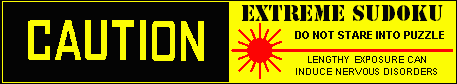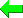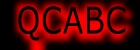Print Version
Solvers
Puzzles
Latest Apps
Str8ts
Other

## Discussion...

Post an idea here...
Your Name or Handle

Your comment, idea or question

 Please enter theletters you see:Remember me

Please keep your comments relevant to this puzzle.
Email addresses are never displayed, but they are required to confirm your comments. To state your solve time use the smaller form at the top right of this page." & vbNewLine & _ Line breaks and paragraphs are automatically converted ? no need to use <p> or <br> tags.

## ... by: ghfick

While I am fairly competent with JE based on David P Bird's compendium, I am far less secure with Exocets [that are not JE].
I am still puzzling about the confirming process with U314. In order to confirm that the target cells contain different base digits, I am still thinking one needs to check every pair of possible base digits.
btw I did look at the solution and it is indeed an Exocet as the pair of true base digits are in the target cells [a different true base digit for each target]

## ... by: ghfick

After staring at this exocet for far too long, I now realize that the target cells contain only base cells. There is no checking required. While there are then no exclusions to the target cells, all the other inferences come into play as noted by Cenoman and numpl_npm. [sigh]

## ... by: Cenoman

Hi numpl_npm,

I agree with you, "false in both mirror nodes" is enough. It is a direct consequence of the pattern definition. My further development was just for helping.

Best regards,
Cenoman

## ... by: Cenoman

Hi Gordon,

Recently, in a thread known of you, as you made some contributions to this, I asked for help to solve hardest puzzles.
forum.enjoysudoku.com/help-to-solve-hardest-puzzles-t34876.html

In this thread, for exocet pattern identification, champagne gave the following advice:
[quote]
Step 1 check that this is an exocet pattern for each digit
a) forces the digit in the base,
b) clear the digit in the target
c) check that you have no solution
[end of quote]

Note that in this process, digits are considered one by one, not by pairs.

As I asked if such a process was not assumptive, champagne answered:
[quote]
{It} is as assumptive as any clearing rule, not less, not more.
point c) is usually an easy step done on the pm of the digit
[end of quote]

So for WU#314, I have checked with basic techniques, digit per digit, that, when forcing the digit in C45 and clearing it in A8 & B2, the puzzle has no solution (I get a contradiction).
Then I focused on solving the puzzle and did not care so much for a formal demo of the pattern identification.

Now your question made me check if point c) is really easy. Here are my findings:
- digit 4, the demo is trivial. +4C45 & -4A8 & -4B2 leave Row A void of 4 after basics.
- digit 1 (resp. 3), the demo is easy. +1C45 & -1A8 & -1B2 (resp. 3) leave Swordfishes of 1 (resp. 3) at EFJ126 and ABD379. Eliminations from the sworfishes leave box 9 void of 1 (resp. 3)
- digit 2, the demo is easy. +2C45 & -2A8 & -2B2 leave a Swordfish of 2 at EFH126 and an X-wing of 2 at AB39. Eliminations from the sworfish and X-wing leave box 6 void of 2

Best regards,
Cenoman

## ... by: numpl_npm

Correct previous post:

And if Y=y, then => Let x, y in AA. And if Y=x, then
either y not in ss or y not in tt. so y in ww and X=y.

## ... by: numpl_npm

'as it false in both "mirror nodes" (A79, B13)', that is enough for me.

9 8 _ | 7 6 _ | 5 Y _
7 X _ | _ 5 _ | _ 9 _
_ _ _ | A A 9 | _ _ 8

5 9 w | u u 6 | s 8 s
_ _ 4 | _ 7 _ | _ _ _
_ _ _ | 3 _ _ | _ _ 5

4 6 w | v v 7 | t 5 t
_ _ _ | _ 1 _ | _ _ 4
_ _ _ | _ _ _ | 2 _ _

Let y which is not 4 in AA.
If 4 in AA, then X=4 and Y=4, but y<>123, so 4 not in AA.

y<>1, y<>3 <-- y in ss and y in tt.
y<>2 <-- y in uu and y in vv.

And if Y=y, then
either y not in ss or y not in tt. so y in ww and X=y.

## ... by: ghfick

Hi Cenoman,
Could you show us how you determined that band exocet? I think you have shown that each base pair combination satisfies the exocet condition by finding chains that demonstrate the target cells have the necessary base candidate. Maybe you have an alternate method? All methods would appear to be rather laborious [and assumptive!], if I am correct here.
Best
Gordon

## ... by: Cenoman

Sorry, should read WU#314 has a band exocet....

## ... by: Cenoman

WU#313 has a band exocet (1234)C45, A8, B2
Warning: I wrote exocet, not Junior Exocet (which it isn't)...

1. Eliminations from the exocet pattern:
* 4 cannot be a true base digit, as it false in both "mirror nodes" (A79, B13); or in other terms, if 4 is assumed True in the base cells C45, it is forced to be True in both target cells A8 & B2. =>-4C45, -4A8, -B2 (4 is also eliminated from the target cells which can contain only true base digits)

** 6 is eliminated from B3 as non-base digit in the second cell of a mirror node (first cell already locked with a non-base digit 7B1) =>-6B3 (B9=6)

=>8 placements + basics
The puzzle is now solved with a turbot-fish and three krakens (or forcing nets as named in Andrew's solver)

2. (7)J9=D9-D3=(7)F2 =>-7J2

3. Kraken cell (12389)G3 =>-8H6
||(123)G3-(123=7)ABD3-F2=(79-8)F57=(8)GJ5
||(8)G3-G7=(8)H7
||(9)G3-G45=(946-5)H4.J45=(59-8)E4.F5=(8)EF6

4. Kraken cell (1367)J8 =>-5H4
||(13)J8-(135=8)J126-GJ5=(89-7)F57=F2-(7=235)H126
||(6)J8-H8=(6)H4
||(7)J8-C8=C7-F7=F2-(7=235)H126

5. Kraken row (2)F1256 =>-5J4; basics to the end
||(2)F1-(2=135)H1.J12
||(27-5)FH2=(5)J2
||(2)F56-(2=4)D5-J5=(4)J4

## ... by: numpl_npm

If D4=1 and C5=3, then A6=4.

9 8 _ | 7 6 X | 5 e _
7 a _ | _ 5 _ | _ 9 _
_ _ _ | b B 9 | _ _ 8

5 9 _ | A _ 6 | f 8 d
_ _ 4 | _ 7 _ | _ _ _
_ _ _ | 3 _ _ | _ _ 5

4 6 _ | _ c 7 | g 5 h
_ _ _ | _ 1 _ | _ _ 4
_ _ _ | _ _ _ | 2 _ _

A=1, B=3.
Either a=3 or a<>3.
If a=3, then b=2, c=2, d=2, e=2, X=4.
If a<>3, then 3 in fd, 3 in gh, e=3, X=4.

## ... by: numpl_npm

If D4=1 and J6=4, then C5=3.

9 8 _ | 7 6 c | 5 h _
7 a _ | b 5 d | _ 9 _
_ _ _ | _ X 9 | _ i 8

5 9 e | A f 6 | _ 8 g
_ _ 4 | _ 7 _ | _ _ _
_ _ _ | 3 _ _ | _ _ 5

4 6 _ | _ _ 7 | _ 5 _
_ _ _ | _ 1 _ | _ _ 4
_ _ _ | _ _ B | 2 _ _

A=1, B=4.
Either a=2 or a<>2.

If a=2, then b=4, cd=18, X=3.
If a<>2, then Either e=2, f=2 or g=2, and
in any case X=3 (if g=2, so h=4, i=2)

## ... by: numpl_npm

9 8 _ | 7 6 _ | 5 _ _
7 f. _ | d 5 _ | _ 9 _
_ _ _ | e X 9 | _ _ 8

5 9 _ | A h 6 | _ 8 _
_ _ 4 | a. 7 _ | _ _ _
_ _ _ | 3 ._ _ | _ _ 5

4 6 _ | b. g 7 | _ 5 _
_ _ _ | c. 1 _ | _ _ 4
_ _ _ | B C _ | 2 _ _

If D4=1 and J4=4, then C5=3.
Because
A=1, B=4. abc=569, d=8, e=2.
Either f=4 or f=4,
in any case X=3 (if f=4, so g=2, h=4).

If D4=1 and J5=4, then C5=3.
Because
A=1, C=4, h=2, X=3.

## ... by: numpl_npm

If E4=1, then A6=4.

9 8 _ | 7 6 X | 5 u _ ~~ A=1, abc=137, de=24, fghi=5689, jd=24.
7 p _ | f. 5 q | _ 9 _ ~~ Either k=2 or m=2.
_ _ _ | j. r. 9 | _ _ 8 ~~

5 9 a | d e 6 | b 8 c .~~ If k=2, then n=2, p=2, qX=14, jr=23, r=3,
_ _ 4 | A 7 _ | _ _ _ ~~ .. 3 in bc, 3 in st, u=3, X=4.
_ _ _ | 3 _ _ | _ _ 5 ~~

4 6 k | g m 7 | s 5 t ~~ If m=2, then e=4, r=3 and either j=2 or j=4,
_ _ _ | h 1 .n | _ _ 4 ~~ .. in any case p=j, p<>3 (if p=2, so e=2, k=2).
_ _ _ | i ._ ._ | 2 _ _ ~~ .. Then 3 in bc, 3 in st, u=3, X=4.

~ ~ ~ ~ ~ ~ ~ ~ ~ ~ ~ ~ In any case X=4.

## ... by: numpl_npm

If C4=1, then A6=4.

9 8 _ | 7 6 X | 5 i ._ ~~ A=1
7 f ._ | _ 5 _ | _ 9 _ ~~ abc=137, de=24,
_ j ._ | A p 9 | _ _ 8 ~~ Either f<>1 or f=1.

5 9 a | d e 6 | b 8 c ~~ If f<>1, then 1 in bc, 1 in gh, i=1, X=4.
_ _ 4 | _ 7 _ | _ _ _ ~~ If f=1, then j=4,
_ _ _ | 3 _ _ | _ _ 5 ~~ .. Either k=2, m=2 or n=2. in any case p=3.

4 6 k | m n 7 | g 5 h ~~ .. And 3 in bc, 3 in gh, i=3, X=4.
_ _ _ | _ 1 _ | _ _ 4
_ _ _ | _ _ _ | 2 _ _ ~~ In any case X=4.

## ... by: numpl_npm

If B4=1, then A6=4.

9 8 . | 7 6 X | 5 u . A=1,
7 p . | A 5 a | q 9 . a=8, bcd=137, ef=24, ghij=5689.
. . . | . r 9 | . . 8 Either k=2 or m=2.
-------- -------- -------
5 9 b | e f 6 | c 8 d If k=2, then n=2, p=2, q=4, X=4.
. . 4 | g 7 . | . . . If m=2, then f=4, r=3 and
. . . | 3 . . | . . 5 Either p=3 or p<>3,
-------- -------- ------- If p=3, then q=4, X=4.
4 6 n | h m 7 | s 5 t If p<>3, then 3 in cd, 3 in st, u=3, X=4.
. . . | i 1 k | . . 4
. . . | j . . | 2 . . In any case X=4.

## ... by: Neil

To Vinny & Manny, I posted a reply to your question on the U313 page.

## ... by: numpl_npm

If B4=1, C4=1 or E4=1, in any case A6=4.
If D4=1, then either J4=4, J5=4 or J6=4, and in any case C5=3.
If D4=1 and C5=3, then A6=4.
In any case A6=4, this digit determines all other digits.

## ... by: Frans Goosens

With trial and error

Combination A9=123 and H8=367

************************************************************
A9=1 H8=3 No solution, Fixed

Calculate 2 digits cells

A3=2 Wrong, Undo calculation
A3=3 Wrong, Undo calculation

All reset to initial position

************************************************************
A9=1 H8=6 No solution, Fixed

Calculate 2 digits cells

A3=2 No solution, Undo calculation
A3=3 No solution, Undo calculation
D5=2 No solution, Undo calculation
D5=4 Wrong, Undo calculation
D5=2 No solution, Fixed
A3=2 Wrong, Undo calculation
A3=3 Wrong, Undo calculation

All reset to initial position

************************************************************
A9=1 H8=7 No solution, Fixed

Calculate 2 digits cells

A3=2 Wrong, Undo calculation
A3=3 Wrong, Undo calculation

All reset to initial position

************************************************************
A9=2 H8=3 No solution, Fixed

Calculate 2 digits cells

A3=1 No solution, Undo calculation
A3=3 Wrong, Undo calculation
A3=1 No solution, Fixed
C5=2 Wrong, Undo calculation
C5=4 No solution, Fixed
D3=3 Wrong, Undo calculation
D3=7 Wrong, Undo calculation

All reset to initial position

************************************************************
A9=2 H8=6 No solution, Fixed

Calculate 2 digits cells

A3=1 No solution, Undo calculation
A3=3 No solution, Undo calculation
D5=2 No solution, Undo calculation
D5=4 No solution, Undo calculation

All reset to initial position

************************************************************
A9=2 H8=7 No solution, Fixed

Calculate 2 digits cells

A3=1 No solution, Undo calculation
A3=3 No solution, Undo calculation
D5=2 Wrong, Undo calculation
D5=4 No solution, Fixed
D7=1 Wrong, Undo calculation
D7=3 No solution, Fixed

All reset to initial position

************************************************************
A9=3 H8=3 No solution, Fixed

Calculate 2 digits cells

A3=1 No solution, Undo calculation
A3=2 No solution, Undo calculation
D5=2 No solution, Undo calculation
D5=4 ( C5=3 ) Solved,

#314---------------------------Solution
980 760 500-------------981 764 523
700 050 090-------------732 851 496
000 009 008-------------645 239 178

590 006 080-------------597 146 382
004 070 000-------------324 578 619
000 300 005-------------816 392 745

460 007 050-------------463 927 851
000 010 004-------------278 615 934
000 000 200-------------159 483 267

Total solving time is: 242 sec.

## Archive

Each week a new 'unsolvable' will be published and the previous will be accessible here from this archive section. If you like very tough puzzles, these are for you.
Share and Enjoy

Page created on 03-May-2011, last modified on 03-May-2011.
All puzzles on this site are trademarked and copyright
and cannot be reproduced without permission.
Copyright Syndicated Puzzles Inc, 2011-2015